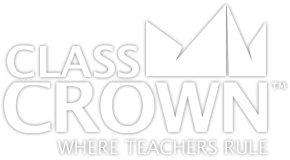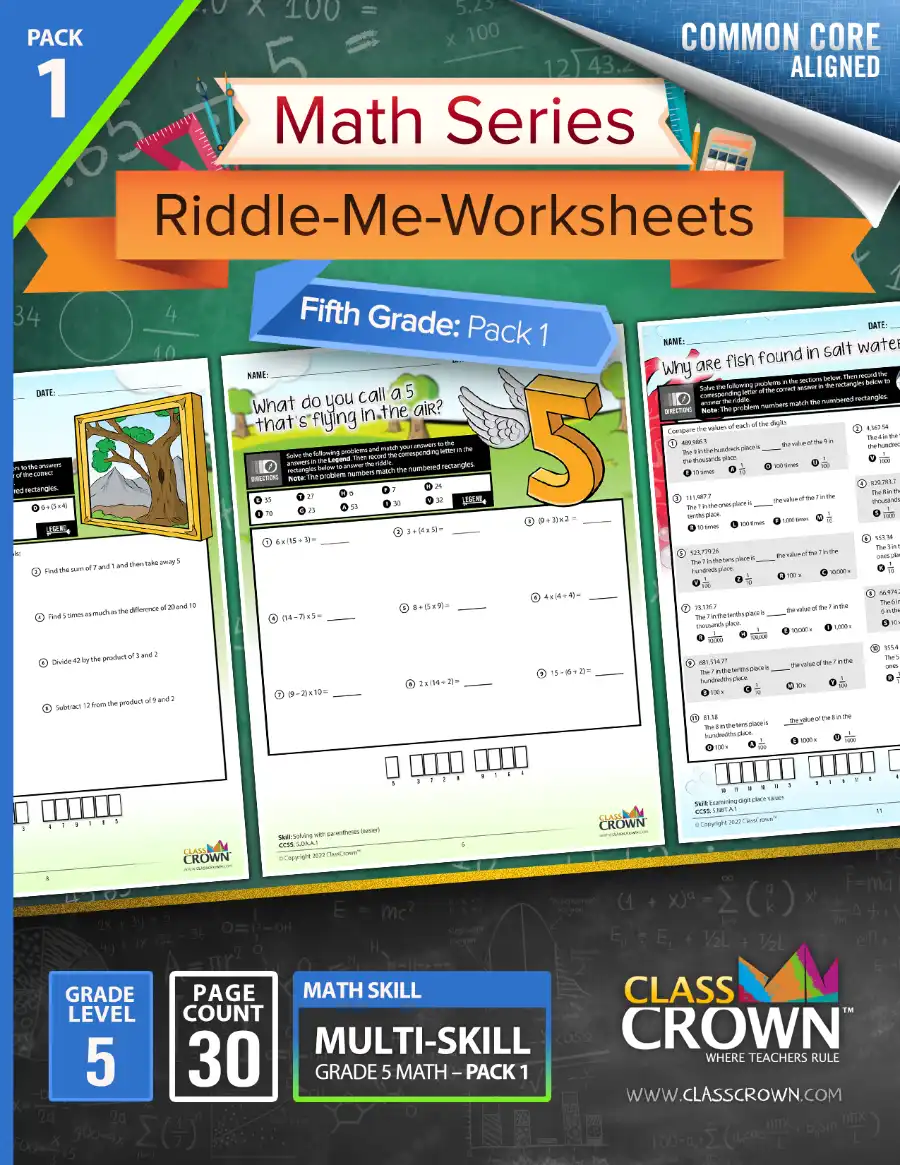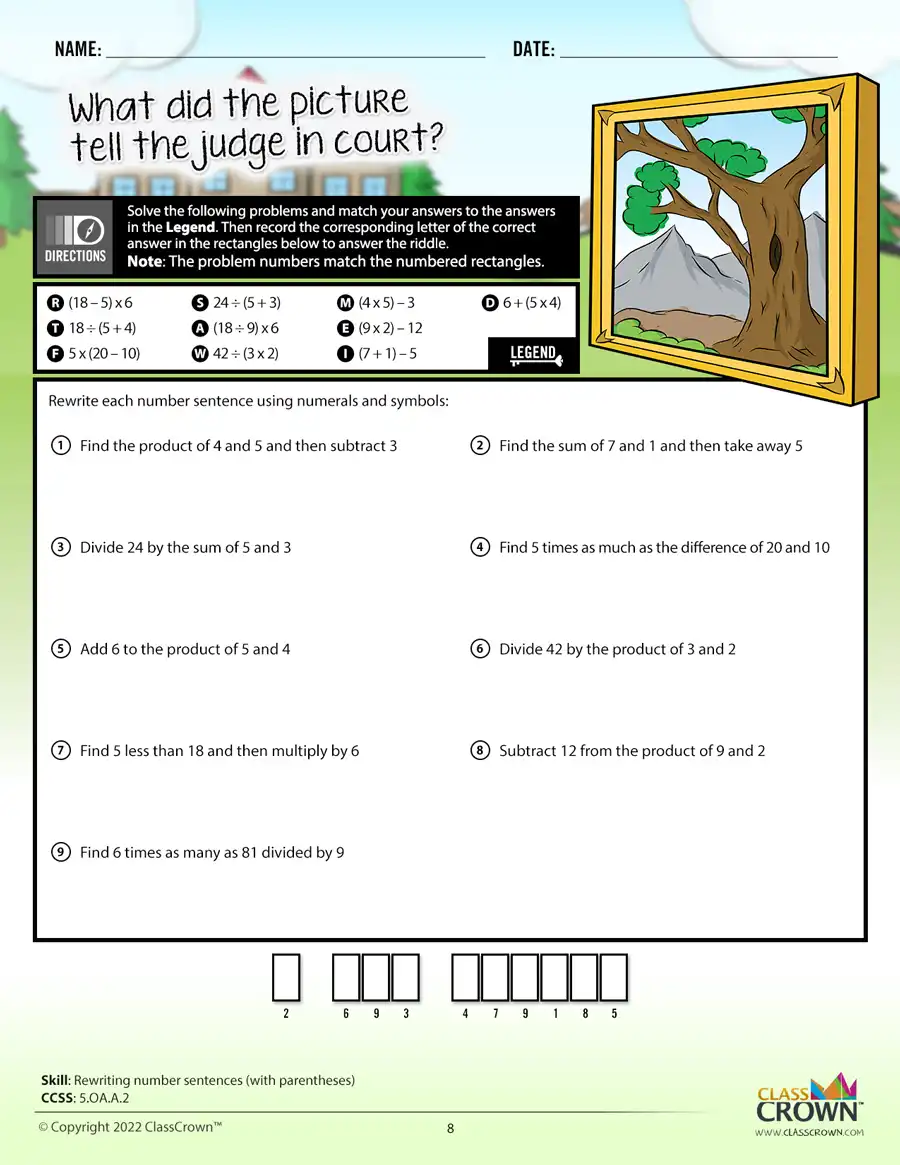Math Worksheets

# 5th Grade Math Worksheets: Pack 1

## Self-checking math worksheets with riddles to keep kids motivated.

Rating: 0/5
(0 Reviews)
5th
WORKSHEET COUNT
30
FORMAT(S)
PDF
Yes
\$8.99
The Details
Description
• Do your students ever complete an entire math worksheet, only to find that they have done most of the problems incorrectly and clearly have a misconception?
• ClassCrown’s Riddle-Me-Worksheets help to prevent this problem by introducing a puzzle aspect to math, giving students immediate feedback as to whether or not they are solving problems correctly. If the answer to the riddle isn't correct, the student knows which problems he's made an error on.
Specifics
• Subject:  Number lines, 4-digit Multiplication, plus more!
• Product Count:  30
• Full Page Count:  51
• File Format(s):  PDF

What's Included?
• Solving with parentheses (easier)
• Solving with parentheses
• Rewriting number sentences (with parentheses)
• Graphing using given rules
• Finding digit values with decimals
• Examining digit place values
• Decimal equation patterns
• Decimal equation patterns
• Multiplying and dividing patterns of ten
• Solving decimal word problems with power of ten
• Understanding multiplying decimals
• Dividing multiples of 10
• Understanding multiplying by 10s and 100s
• Understanding multiplying by 10s, 100s, and 1000s
• Converting decimals from word to numeric form
• Converting expanded notation to numeric form with decimals
• Converting expanded notation to numeric form with decimals
• Converting numeric form to expanded notation with decimals
• Converting numeric form to word form with decimals
• Comparing decimals (thousandths)
• Ordering decimals
• Rounding decimals
• Multiplying 3-digit by 2-digit
• Multiplying 3-digit by 2-digit
• Multiplying 4-digit by 2-digit
• Multiplying 4-digit by 2-digit
• Multiplying multi-digit whole number word problems
• Dividing 4-digit by 2-digit with remainder
• Dividing 3-digit by 2-digit with remainder
• Dividing 4-digit by 2-digit with remainder
CCSS: 5.OA.A.1, 5.OA.A.2, 5.OA.A.3, 5.NBT.A.1, 5.NBT.A.2, 5.NBT.A.3, 5.NBT.A.B, 5.NBT.A.3.B, 5.NBT.A.4, 5.NBT.B.5, 5.NBT.B.6
Reviews
Overall Rating: 0/5
(Showing 0 of 0)
See More Reviews @ TpTSelf Checking

Our math worksheets introduce a puzzle aspect to math, giving students immediate feedback as to whether or not they are solving problems correctly. If the answer to the riddle isn't spelled correctly, the student knows which problems he's made an error on.Fun Puzzle AspectImmediate Feedback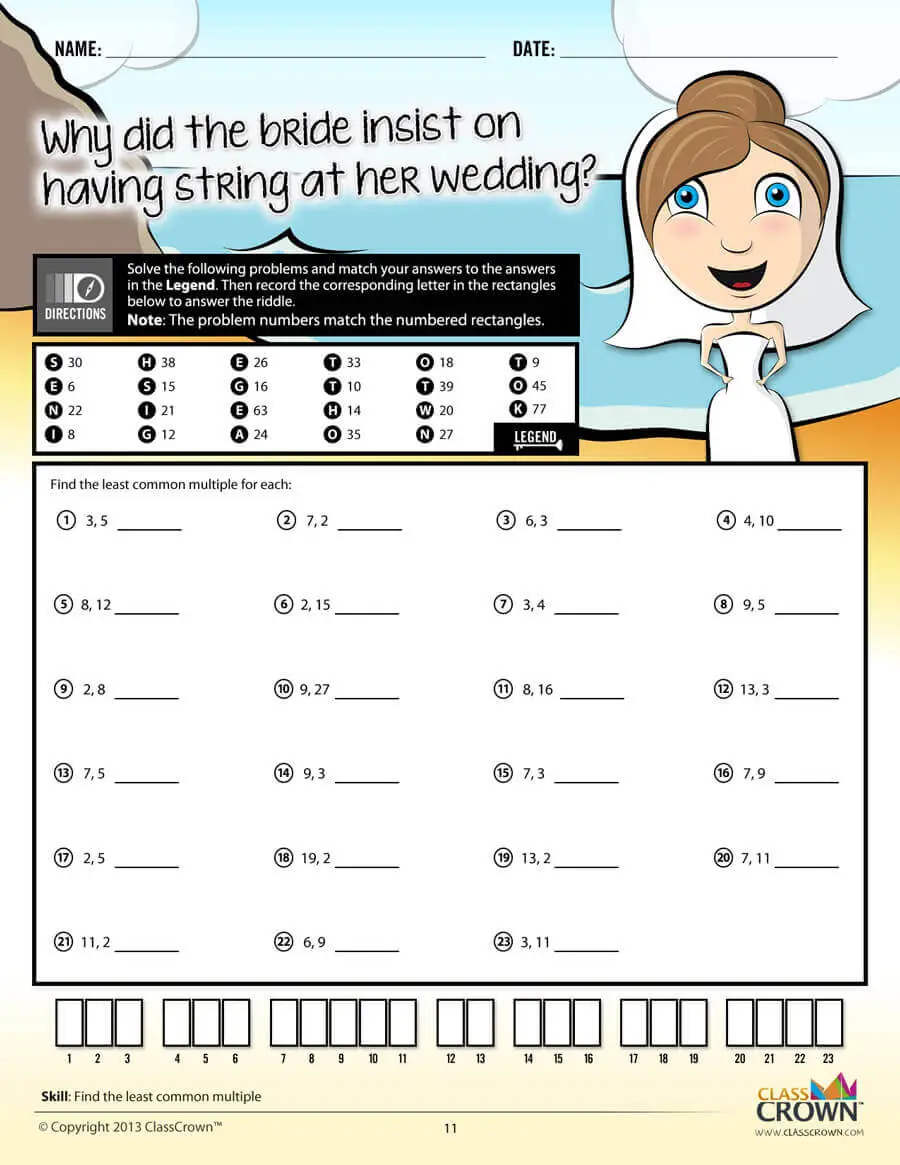Problem Solving Motivation

Each math worksheet contains a riddle that the student solves by completeing all the problems on the worksheet. This keeps kids motivated to complete each problem so that they can find the answer to the riddle.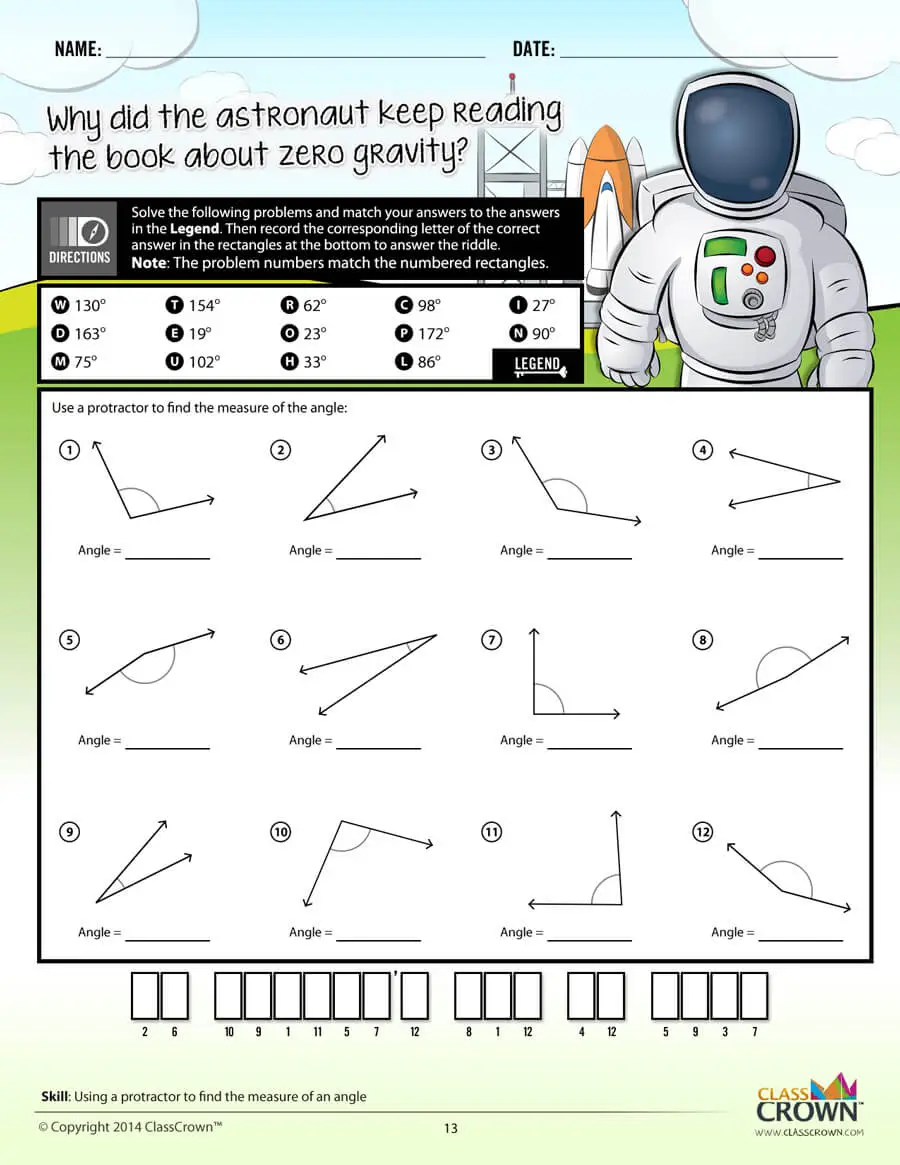Common Core Aligned

All our math worksheet packs are designed with Common Core in mind. That way you don’t have to worry about whether your math ciriculum is aligned or not when you incorpoate ClassCrown Riddle-Me-Worksheets in your lesson plans.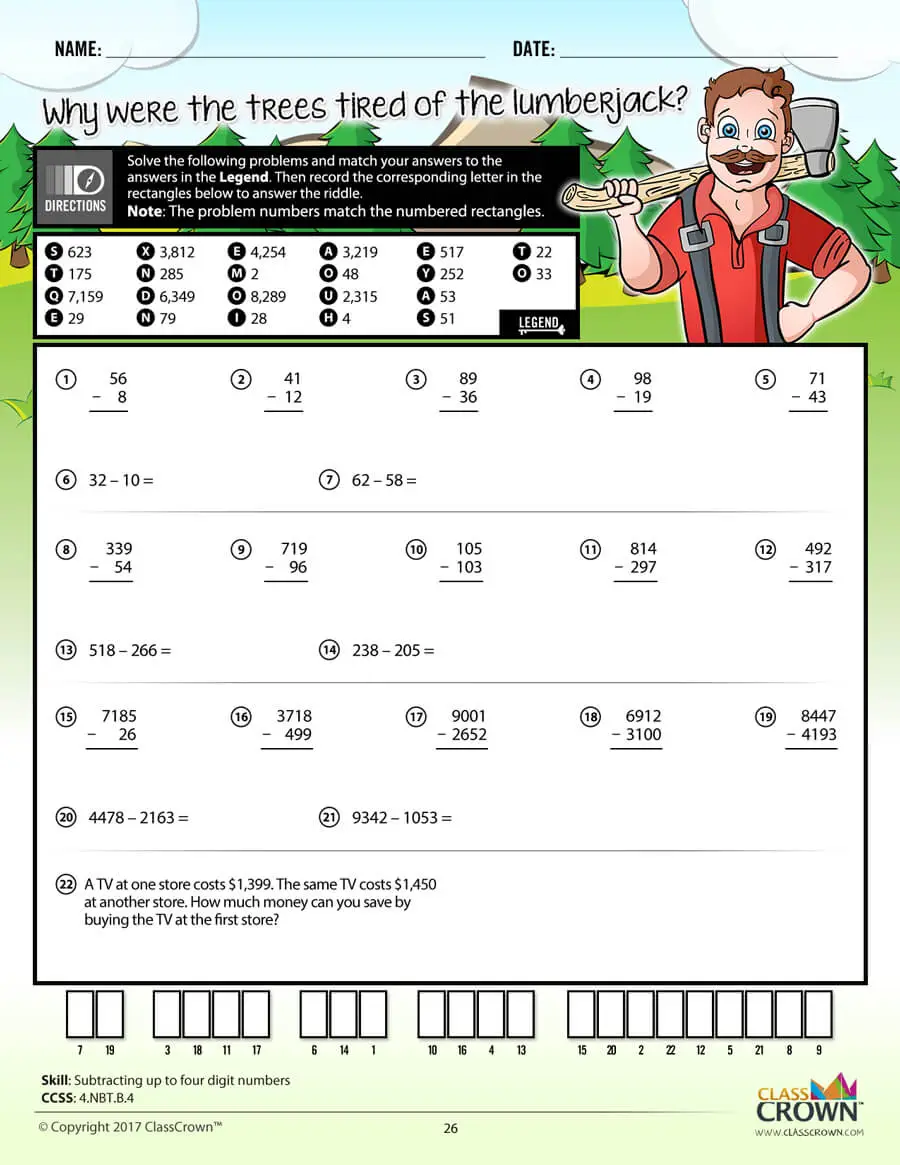High Quality Design

Each page of our math worksheets has been produced in high resolution at 144 dpi and designed in full, vibrant color for maximum quality. They look stunning whether you are printing in color or black and white.High Resolution
(144 dpi)Stunning Color & Clarity